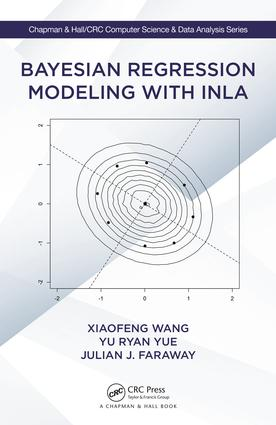Bayesian Regression Modeling with INLA

1st Edition

Chapman and Hall/CRC

312 pages

Purchasing Options:\$ = USD
Hardback: 9781498727259
pub: 2018-02-16
SAVE ~\$18.79
\$93.95
\$75.16
x
eBook (VitalSource) : 9781351165761
pub: 2018-01-29
from \$44.98

FREE Standard Shipping!

Description

INLA stands for Integrated Nested Laplace Approximations, which is a new method for fitting a broad class of Bayesian regression models. No samples of the posterior marginal distributions need to be drawn using INLA, so it is a computationally convenient alternative to Markov chain Monte Carlo (MCMC), the standard tool for Bayesian inference.

Bayesian Regression Modeling with INLA covers a wide range of modern regression models and focuses on the INLA technique for building Bayesian models using real-world data and assessing their validity. A key theme throughout the book is that it makes sense to demonstrate the interplay of theory and practice with reproducible studies. Complete R commands are provided for each example, and a supporting website holds all of the data described in the book. An R package including the data and additional functions in the book is available to download.

The book is aimed at readers who have a basic knowledge of statistical theory and Bayesian methodology. It gets readers up to date on the latest in Bayesian inference using INLA and prepares them for sophisticated, real-world work.

Xiaofeng Wang is Professor of Medicine and Biostatistics at the Cleveland Clinic Lerner College of Medicine of Case Western Reserve University and a Full Staff in the Department of Quantitative Health Sciences at Cleveland Clinic.

Yu Ryan Yue is Associate Professor of Statistics in the Paul H. Chook Department of Information Systems and Statistics at Baruch College, The City University of New York.

Julian J. Faraway is Professor of Statistics in the Department of Mathematical Sciences at the University of Bath.

Reviews

"The book focuses on regression models with R-INLA and it will be of interest to a wide audience. INLA is becoming a very popular method for approximate Bayesian inference and it is being applied to many problems in many different fields. This book will be of interest not only to statisticians but also to applied researchers in other disciplines interested in Bayesian inference. This book can probably be used as a reference book for research and as a textbook at graduate level."

~Virgilio Gómez-Rubio,University of Castilla-La Mancha

"This is a well-written book on an important subject, for which there is a lack of good introductory material. The tutorial-style works nicely, and they have an excellent set of examples. They manage to do a practical introduction with just the right amount of theory background…The book should be very useful to scientists who want to analyze data using regression models. INLA allows users to fit Bayesian models quickly and without too much programming effort, and it has been used successfully in many applications. The book is written in a tutorial style, while explaining the basics of the needed theory very well, so it could serve both as a reference or textbook…The book is well written and technically correct."

~Egil Ferkingstad, deCode genetics

"The authors have done a great job of not over-doing the technical details, thereby making the presentation accessible to a broader audience beyond the statistics world…It covers many contemporary parametric, nonparametric, and semiparametric methods that applied scientists from many fields use in modern research."

~Adam Branscum, Oregon State University

1.Introduction

Quick Start

Hubble’s Law

Standard Analysis

Bayesian Analysis

INLA

Bayes Theory

Prior and Posterior Distributions

Model Checking

Model Selection

Hypothesis testing

Bayesian Computation

Exact

Sampling

Approximation

2.Theory of INLA

Latent Gaussian Models (LGMs)

Gaussian Markov Random Fields (GMRFs)

Laplace Approximation and INLA

INLA Problems

Extensions

3.Bayesian Linear Regression

Introduction

Bayesian Inference for Linear Regression

Prediction

Model Selection and Checking

Model Selection by DIC

Posterior Predictive Model Checking

Cross-validation Model Checking

Bayesian Residual Analysis

Robust Regression

Analysis of Variance

Ridge Regression for Multicollinearity

Regression with Autoregressive Errors

4.Generalized Linear Models

GLMs

Binary Responses

Count Responses

Poisson Regression

Negative binomial regression

Modeling Rates

Gamma Regression for Skewed Data

Proportional Responses

Modeling Zero-inflated Data

5.Linear Mixed and Generalized Linear Mixed Models

Linear Mixed Models

Single Random Effect

Choice of Priors

Random Effects

Longitudinal Data

Random Intercept

Random Slope and Intercept

Prediction

Classical Z-matrix Model

Ridge Regression Revisited

Generalized Linear Mixed Models

Poisson GLMM

Binary GLMM

Improving the Approximation

6.Survival Analysis

Introduction

Semiparametric Models

Piecewise Constant Baseline Hazard Models

Stratified Proportional Hazards Models

Accelerated Failure Time Models

Model Diagnosis

Interval Censored Data

Frailty Models

Joint Modeling of Longitudinal and Time-to-event Data

7.Random Walk Models for Smoothing Methods

Introduction

Smoothing Splines

Random Walk (RW) Priors for Equally-spaced Locations

Choice of Priors on s e and sf

Random Walk Models for Non-equally Spaced Locations

Thin-plate Splines

Thin-plate Splines on Regular Lattices

Thin-plate Splines at Irregularly-spaced Locations

Besag Spatial Model

Penalized Regression Splines (P-splines)

Generalized Nonparametric Regression Models

Excursion Set with Uncertainty

8.Gaussian Process Regression

Introduction

Penalized Complexity Priors

Credible Bands for Smoothness

Non-stationary Fields

Interpolation with Uncertainty

Survival Response

Binary response

Count response

Generalized Additive Mixed Models

10.Errors-in-Variables Regression

Introduction

Classical Errors-in-Variables Models

A simple linear model with heteroscedastic errors-invariables

A general exposure model with replicated measurements

Berkson Errors-in-Variables Models

11.Miscellaneous Topics in INLA

Splines as a Mixed Model

Truncated Power Basis Splines

O’Sullivan Splines

Example: Canadian Income Data

Analysis of Variance for Functional Data

Extreme Values

Density Estimation using INLA

Appendix A Installation

Appendix B Uninformative Priors in Linear Regression

Index

Xiaofeng Wang is Professor of Medicine and Biostatistics at the Cleveland Clinic Lerner College of Medicine of Case Western Reserve University and a Full Staff in the Department of Quantitative Health Sciences at Cleveland Clinic.

Yu Ryan Yue is Associate Professor of Statistics in the Paul H. Chook Department of Information Systems and Statistics at Baruch College, The City University of New York.

Julian J. Faraway is Professor of Statistics in the Department of Mathematical Sciences at the University of Bath.